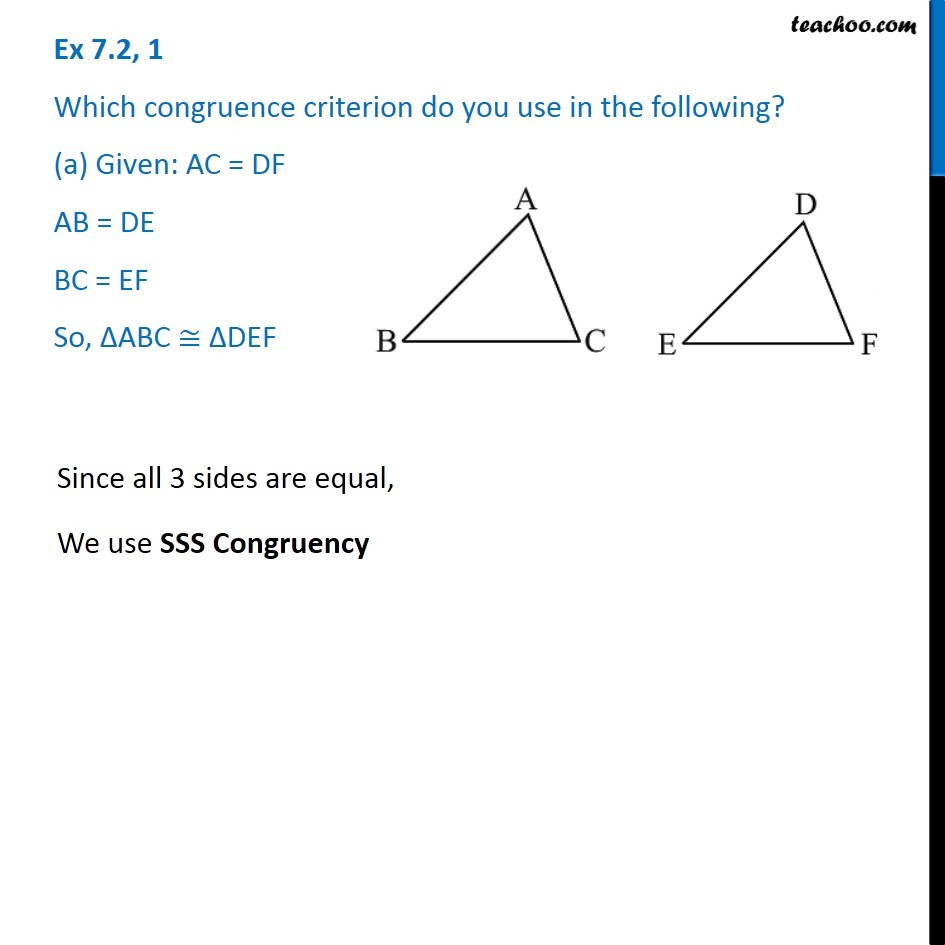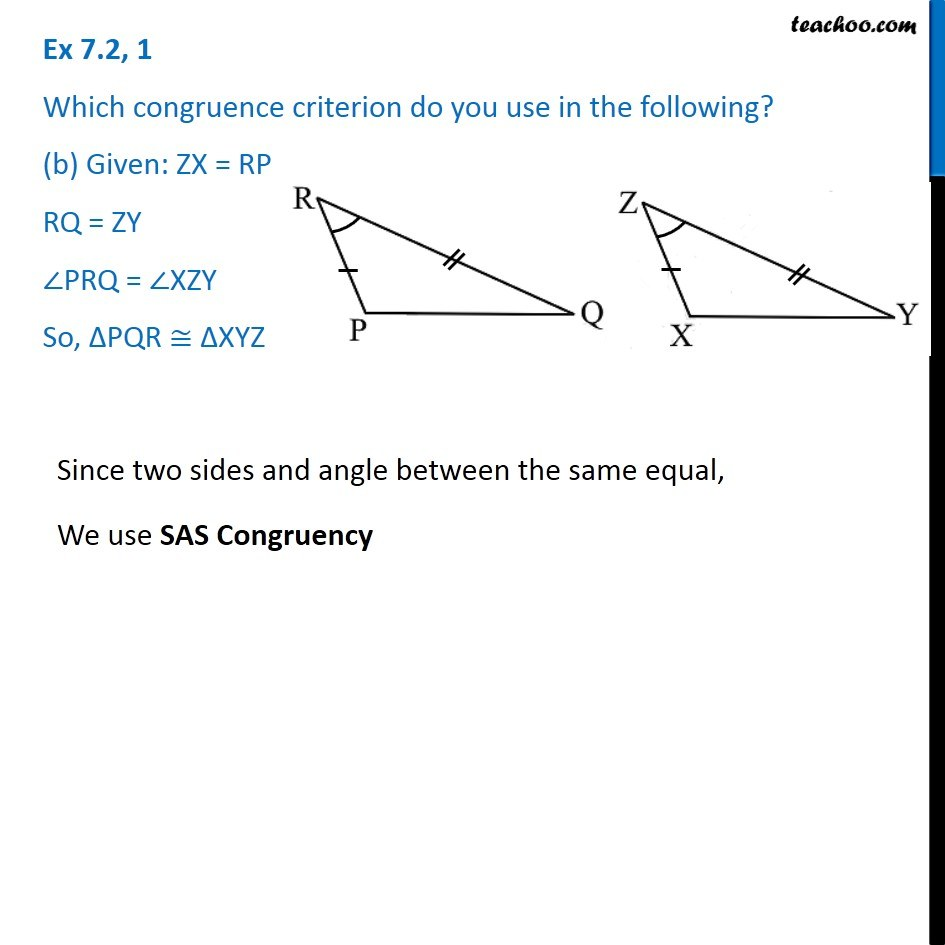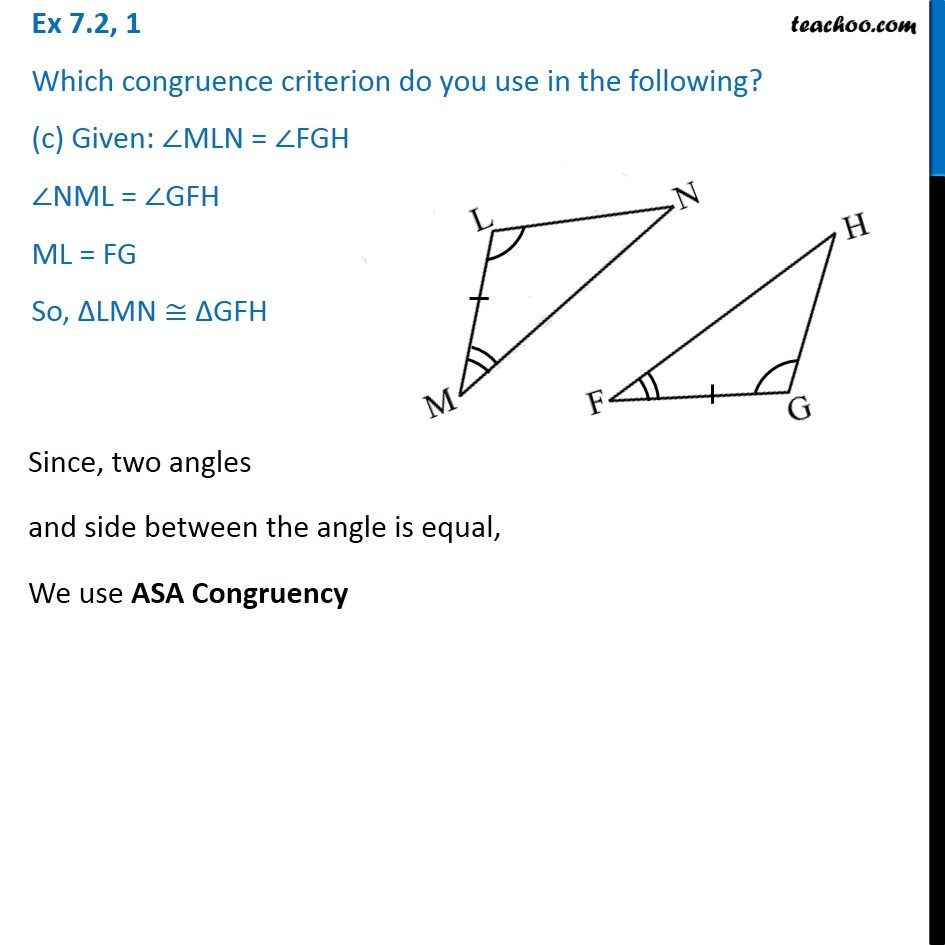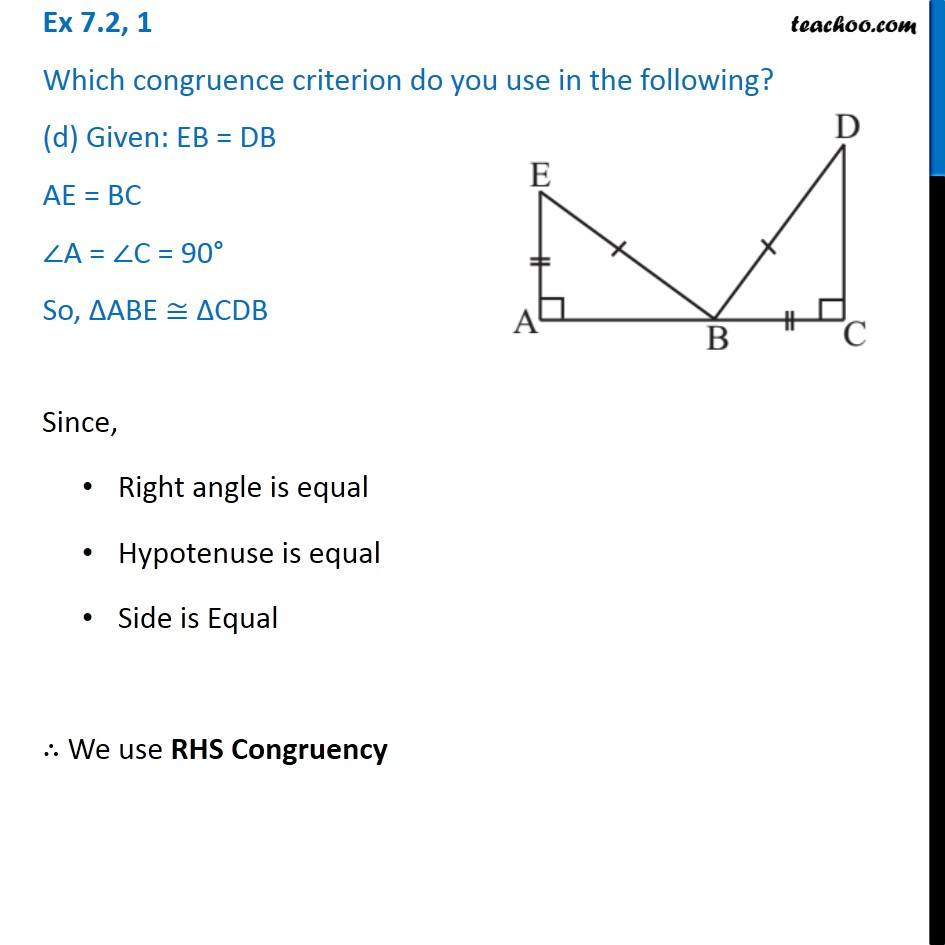1. Chapter 7 Class 7 Congruence of Triangles
2. Concept wise
3. Congruency Criteria Mix questions

Transcript

Ex 7.2, 1 Which congruence criterion do you use in the following? (a) Given: AC = DF AB = DE BC = EF So, ∆ABC ≅ ∆DEF Since all 3 sides are equal, We use SSS Congruency Ex 7.2, 1 Which congruence criterion do you use in the following? (b) Given: ZX = RP RQ = ZY ∠PRQ = ∠XZY So, ∆PQR ≅ ∆XYZ Since two sides and angle between the same equal, We use SAS Congruency Ex 7.2, 1 Which congruence criterion do you use in the following? (c) Given: ∠MLN = ∠FGH ∠NML = ∠GFH ML = FG So, ∆LMN ≅ ∆GFH Since, two angles and side between the angle is equal, We use ASA Congruency Ex 7.2, 1 Which congruence criterion do you use in the following? (d) Given: EB = DB AE = BC ∠A = ∠C = 90° So, ∆ABE ≅ ∆CDB Since, Right angle is equal Hypotenuse is equal Side is Equal ∴ We use RHS Congruency

Congruency Criteria Mix questions